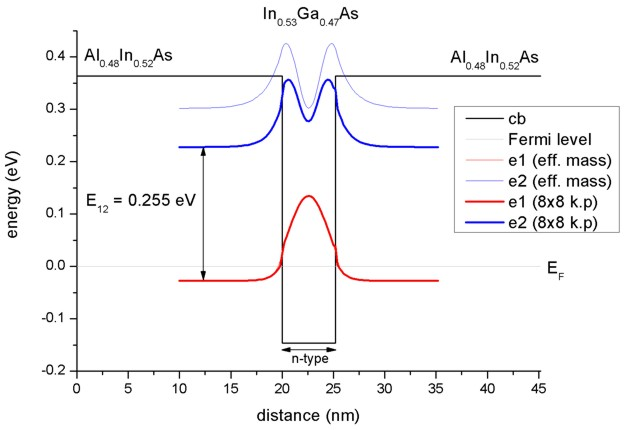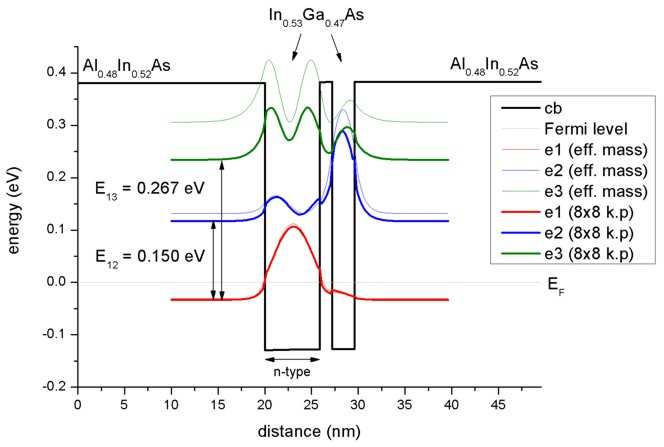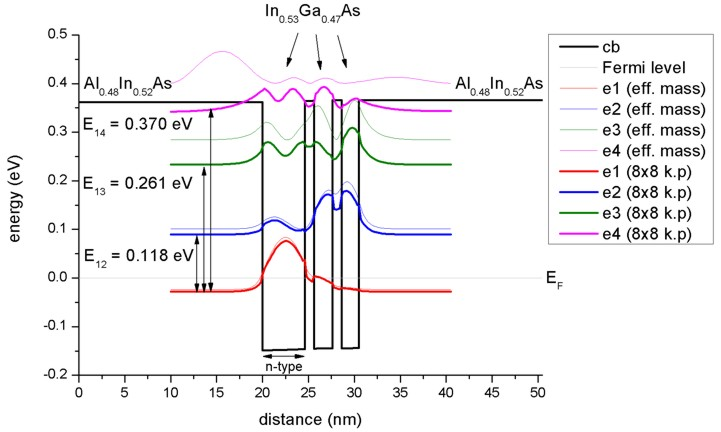www.nextnano.com/documentation/nextnanoplus_tutorials/

# nextnano3 - Tutorial

## Intersubband transitions in InGaAs/AlInAs multiple quantum well systems

Authors: Stefan Birner

```-> 1DSirtoriPRB1994_OneWell_sg_self-consistent_nn3.in           / *_nnp.in  -  ```(single-band effective mass approximation)``` -> 1DSirtoriPRB1994_OneWell_sg_quantum-only_nn3.in              / *_nnp.in  -  ```(single-band effective mass approximation)``` -> 1DSirtoriPRB1994_OneWell_kp_quantum-only_nn3.in              / *_nnp.in  -  ```(8-band k.p)``` -> 1DSirtoriPRB1994_TwoCoupledWells_sg_self-consistent_nn3.in   / *_nnp.in  -  ```(single-band effective mass approximation)``` -> 1DSirtoriPRB1994_TwoCoupledWells_sg_quantum-only_nn3.in      / *_nnp.in  -  ```(single-band effective mass approximation)``` -> 1DSirtoriPRB1994_TwoCoupledWells_kp_quantum-only_nn3.in      / *_nnp.in  -  ```(8-band k.p)``` -> 1DSirtoriPRB1994_ThreeCoupledWells_sg_self-consistent_nn3.in / *_nnp.in  -  ```(single-band effective mass approximation)``` -> 1DSirtoriPRB1994_ThreeCoupledWells_sg_quantum-only_nn3.in    / *_nnp.in  -  ```(single-band effective mass approximation)``` -> 1DSirtoriPRB1994_ThreeCoupledWells_kp_quantum-only_nn3.in    / *_nnp.in  -  ```(8-band k.p)

## Intersubband transitions in InGaAs/AlInAs multiple quantum well systems

This tutorial aims to reproduce Figs. 4 and 5 of
C. Sirtori, F. Capasso, J. Faist
Nonparabolicity and a sum rule associated with bound-to-bound and bound-to-continuum intersubband transitions in quantum wells

Physical Review B 50 (12), 8663 (1994)

This tutorial nicely demonstrates that for the ground state energy the single-band effective mass approximation is sufficient whereas for the higher lying states a nonparabolic model, like the 8-band k.p approximation, is necessary.
This is important for e.g. quantum cascade lasers where higher lying states have a dominant role.

### Step1: Layer sequence

We investigate three structures:
a) a single quantum well
b) two coupled quantum wells
c) three coupled quantum wells

### Step 2: Material parameters

We use In0.53Ga0.47As as the quantum well material and Al0.48In0.52As as the barrier material. Both materials are lattice matched to the substrate material InP. Thus we assume that the InGaAs and AlInAs layers are unstrained with respect to the InP substrate.

The paper
C. Sirtori, F. Capasso, J. Faist
Nonparabolicity and a sum rule associated with bound-to-bound and bound-to-continuum intersubband transitions in quantum wells

Physical Review B 50 (12), 8663 (1994)
lists the following material parameters:

 conduction band offset Al0.48In0.52As/In0.53Ga0.47As `0.510 eV` conduction band effective mass (In0.53Ga0.47As) `0.043 m``0` conduction band effective mass (Al0.48In0.52As) `0.072 m``0`

The temperature is set to 10 Kelvin.

### Step 3: Method

Single-band effective mass approximation

Because our structure is doped, we have to solve the single-band Schrödinger-Poisson equation self-consistently.
The doping is such that the electron ground state is below the Fermi level and all other states are far away from the Fermi level, i.e. only the ground state is occupied and contributes to the charge density.

``` \$simulation-flow-control   flow-scheme      = 2   raw-potential-in = no  \$quantum-model-electrons   ...   model-name                     = effective-mass   number-of-eigenvalues-per-band = 3     ! ```Note: Single-band eigenstates are two-fold spin degenerate.

The Fermi level is always equal to 0 eV in our simulations and the band profile is shifted accordingly to meet this requirement.

8-band k.p approximation

Old version of this tutorial:

Becauce both, the single-band and the 8-band k.p ground state energy and the corresponding wave functions are almost identical, we can read in the self-consistently calculated electrostatic potential of the single-band approximation and calculate for this potential the 8-band k.p eigenstates and wave functions for k|| = 0.

``` \$simulation-flow-control  flow-scheme      = 3  raw-directory-in = raw_data/  raw-potential-in = yes \$quantum-model-electrons  ...  model-name                     = 8x8kp  number-of-eigenvalues-per-band = 6     ! ```Note: One k.p eigenstate for each spin component.

New version of this tutorial:

We provide input files for:
a) self-consistent single-band Schrödinger equation (because the structure is doped)
b) single-band Schrödinger equation (without self-consistency)
c) 8-band k.p single-band Schrödinger equation (without self-consistency)

For a), although the structure is doped, the band bending is very small. Thus we omit for the single-band / k.p comparison in b) and c) the self-consistent cycle.

### Step 4: Results

• Single quantum well

The following figure shows the lowest two electron eigenstates for an In0.53Ga0.47As/Al0.48In0.52As quantum well structure calculated with single-band effective mass approximation and with a nonparabolic 8-band k.p model. The energies (and wave functions, i.e. psi²) for the ground state are identical in both models but the second eigenstate differs substantially. Clearly the single-band model leads to an energy which is far too high for the upper state. Our calculated value for the intersubband transition energy E12 of 255 meV compares well with both, the calculated value of Sirtori et al. (258 meV) and their measured value (compare with absorption curve in Fig. 4 of their paper).The calculated intersubband dipole moments are:
z12 = 1.55 nm (single-band)
z12 =  ... nm (8-band k.p)
(Sirtori's paper: 1.53 nm (exp.), 1.48 nm (th.)).

The influence of doping on the eigenenergies is negligible (smaller than 1 meV).
• Two coupled quantum wells

The following figure shows the lowest three electron eigenstates for an In0.53Ga0.47As/Al0.48In0.52As double quantum well structure calculated with single-band effective mass approximation and with a nonparabolic 8-band k.p model. The energies (and wave functions, i.e. psi²) for the ground state are very similar in both models but the second and especially the third eigenstate differ substantially. Clearly the single-band model leads to energies which are far too high for the higher lying states. Our calculated values for the intersubband transition energies E12 = 150 meV and E13 = 267 meV compare well with both, the calculated values of Sirtori et al. (150 meV and 271 meV) and their measured values (compare with absorption curve in Fig. 5 (a) of their paper).The calculated intersubband dipole moments are:
z12 = 1.61 nm (single-band)
z13 = 1.11 nm (single-band)
z12 = ... nm (8-band k.p)
z13 = ... nm (8-band k.p)
(Sirtori's paper: z12 = 1.64 nm (exp.), z12 = 1.65 nm (th.))

The influence of doping on the eigenenergies is almost negligible (between 0 and 2 meV).
• Three coupled quantum wells

The following figure shows the lowest four electron eigenstates for an In0.53Ga0.47As/Al0.48In0.52As triple quantum well structure calculated with single-band effective mass approximation and with a nonparabolic 8-band k.p model. The energies (and wave functions, i.e. psi²) for the ground state are similar in both models but the second and especially the third and forth eigenstates differ substantially. Clearly the single-band model leads to energies which are far too high for the higher lying states. Our calculated values for the intersubband transition energies E12 = 118 meV, E13 = 261 and E14 = 370 meV compare well with both, the calculated values of Sirtori et al. (116 meV, 257 meV and 368 meV) and their measured values (compare with absorption curve in Fig. 5 (b) of their paper).The calculated intersubband dipole moments are:
z12 = 1.81 nm (single-band)
z13 =   0.77 nm(single-band)
z14 =   0.30 nm(single-band)
z12 =  ... nm (8-band k.p)
z13 =  ... nm (8-band k.p)
z14 =  ... nm (8-band k.p)
(Sirtori's paper: z12 = 1.86 nm (exp.), z12 = 1.84 nm (th.))

The influence of doping on the eigenenergies is almost negligible (between 0 and 4 meV).